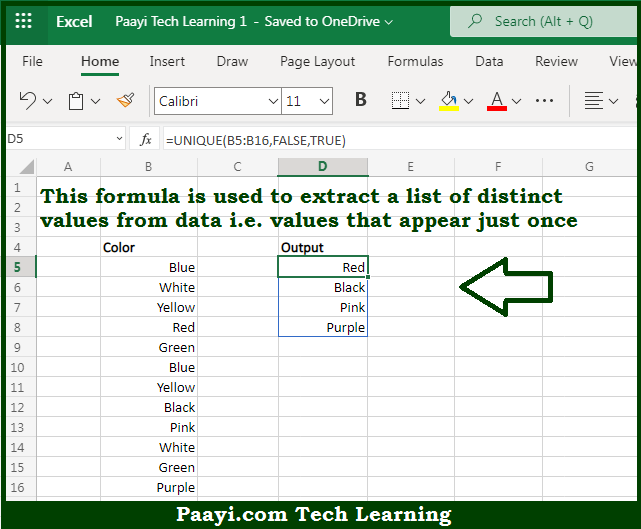# Learn How to Extract Distinct Values in Microsoft Excel

Written by | 0 Comments | 522 Views

In this article, you will learn how to evaluate things in Dynamic Arrays with formulas in Microsoft Excel using a single/combination(s) of functions. You will also know How to Extract Distinct Values and see the generic formula.

How to Extract Distinct Values in Microsoft Excel

The main purpose of this formula is to extract a list of distinct values from a set of data that is values that appear just once. Here we will learn how to extract distinct values from the given data set in the workbook in Microsoft Excel. That implies, with the help of a formula based on the UNIQUE function you can able to extract a list of distinct values from a set of data that is values that appear just once. So, with the help of this formula, you can able to extract distinct values from the given data set in the workbook in Microsoft Excel.

General Formula to Extract Distinct Values

=UNIQUE(data,FALSE,TRUE)

The Explanation for the Extract Distinct ValuesSo we know that with the help of the given formula above you can able to extract a list of distinct values from a set of data that is values that appear just once. Here we will learn how to extract distinct values from the given data set in the workbook in Microsoft Excel. As we know that the UNIQUE function is used to extract a list of unique values, which implies values that appear one or more times in the source data. It should be noted, the UNIQUE function has an optional third argument, called "occurs_once" that, when set to TRUE, will cause UNIQUE to return only values that appear once in the data. So, with the help of this formula, you can able to extract a list of distinct values from a set of data that is values that appear just once. Here we will learn how to extract distinct values from the given data set in the workbook in Microsoft Excel.